# 4 3 Atomic Mass Atomic Mass Isotopes Atomic

• Slides: 15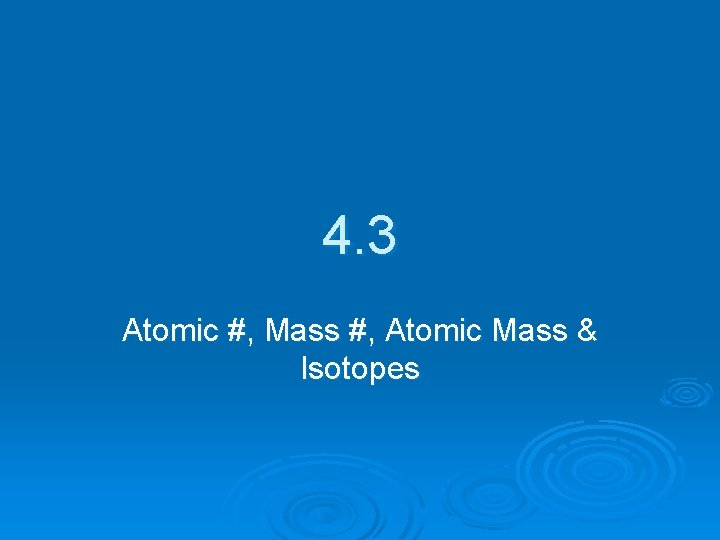4. 3 Atomic #, Mass #, Atomic Mass & Isotopes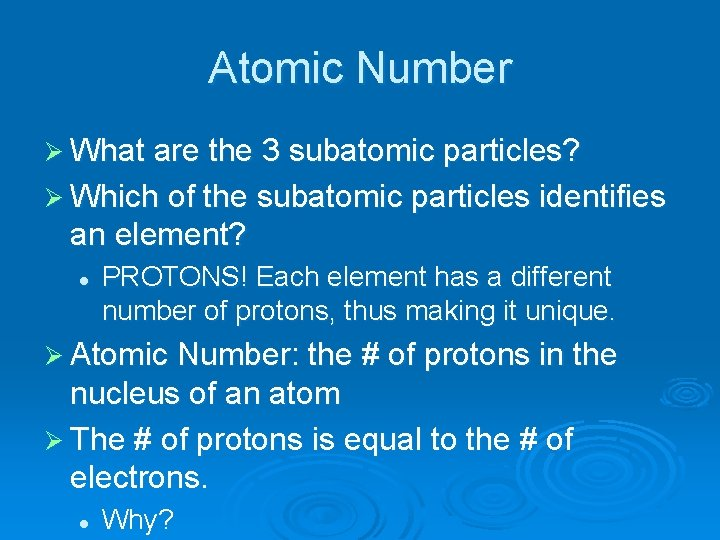Atomic Number Ø What are the 3 subatomic particles? Ø Which of the subatomic particles identifies an element? l PROTONS! Each element has a different number of protons, thus making it unique. Ø Atomic Number: the # of protons in the nucleus of an atom Ø The # of protons is equal to the # of electrons. l Why?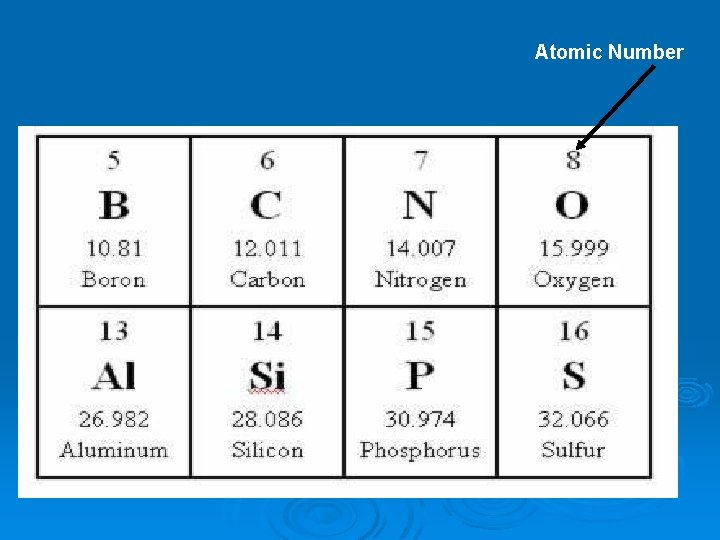Atomic Number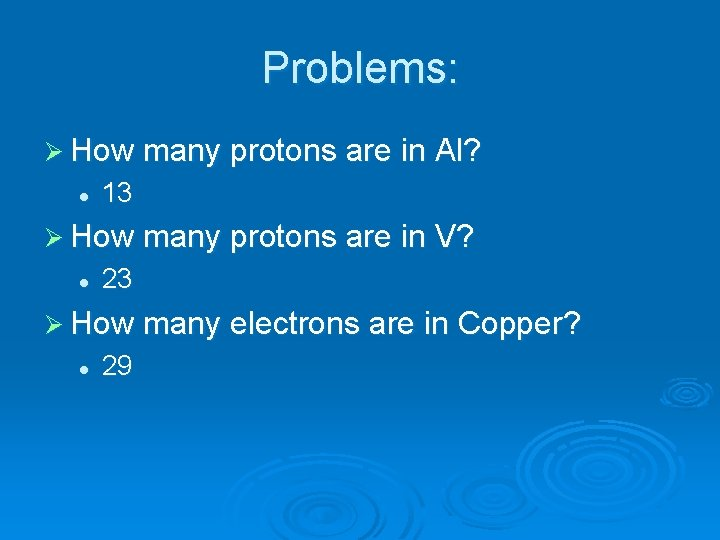Problems: Ø How many protons are in Al? l 13 Ø How many protons are in V? l 23 Ø How many electrons are in Copper? l 29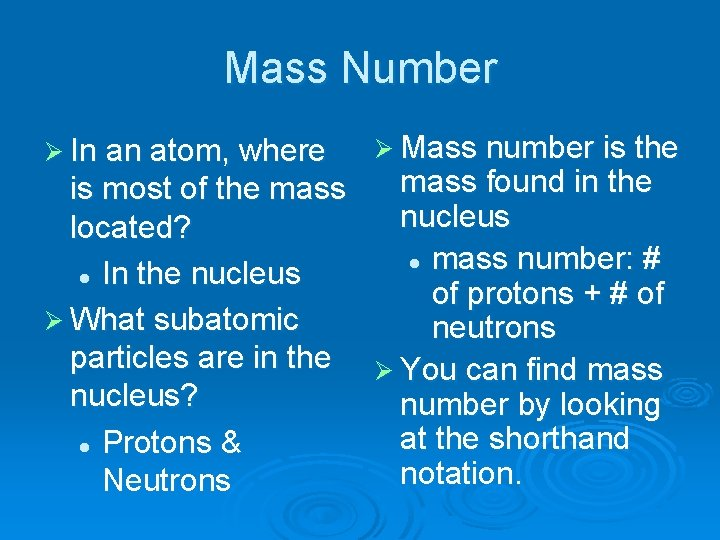Mass Number Ø In an atom, where Ø Mass number is the mass found in the is most of the mass nucleus located? l mass number: # l In the nucleus of protons + # of Ø What subatomic neutrons particles are in the Ø You can find mass nucleus? number by looking at the shorthand l Protons & notation. Neutrons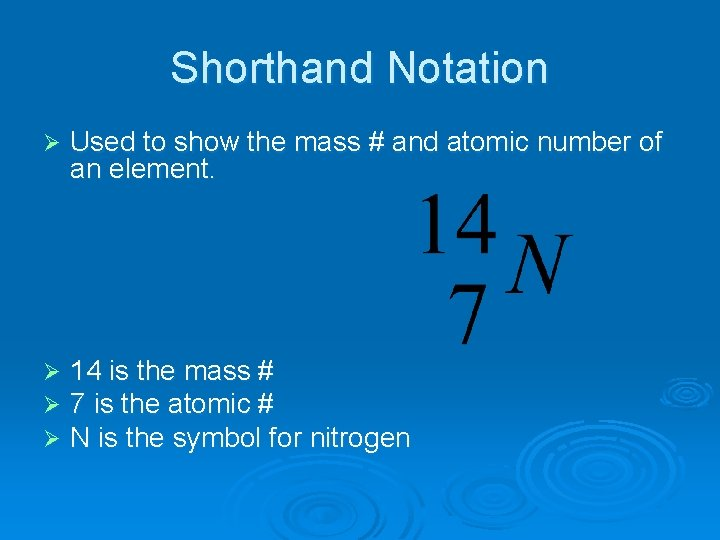Shorthand Notation Ø Used to show the mass # and atomic number of an element. Ø Ø Ø 14 is the mass # 7 is the atomic # N is the symbol for nitrogenShorthand Notation Ø Can also be written as: element-mass # l Examples Nitrogen-14, Nitrogen-15, Nitrogen 16 Ø How many neutrons are in each of the above elements? Ø What is the shorthand notation for -197? gold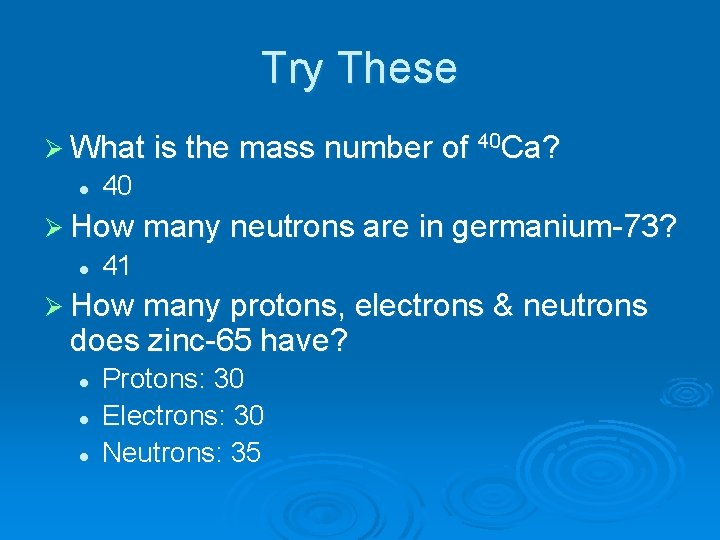Try These Ø What is the mass number of 40 Ca? l 40 Ø How many neutrons are in germanium-73? l 41 Ø How many protons, electrons & neutrons does zinc-65 have? l l l Protons: 30 Electrons: 30 Neutrons: 35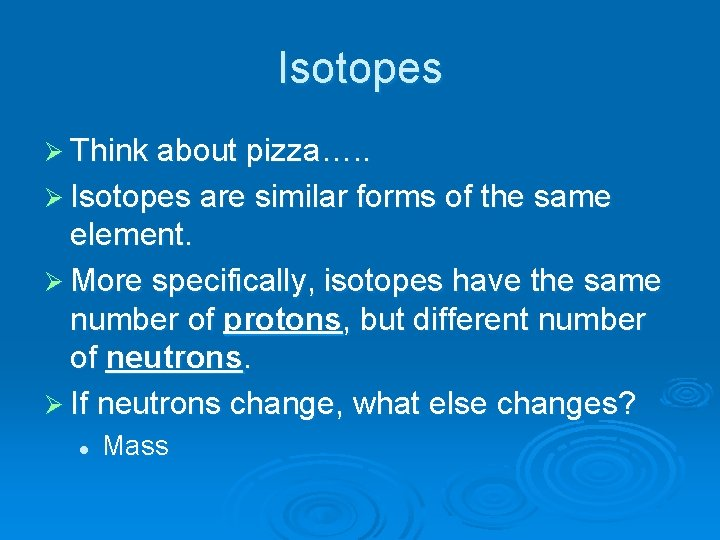Isotopes Ø Think about pizza…. . Ø Isotopes are similar forms of the same element. Ø More specifically, isotopes have the same number of protons, but different number of neutrons. Ø If neutrons change, what else changes? l MassAtomic Mass Ø We must take in account all of the isotopes in order to get an accurate mass. Ø The mass on the PT is a weighted average of all the naturally occurring isotopes of an element. Ø This is called atomic mass.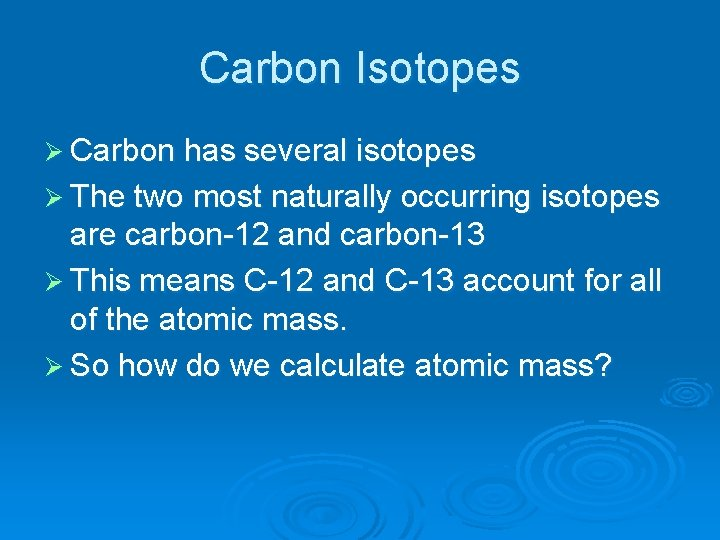Carbon Isotopes Ø Carbon has several isotopes Ø The two most naturally occurring isotopes are carbon-12 and carbon-13 Ø This means C-12 and C-13 account for all of the atomic mass. Ø So how do we calculate atomic mass?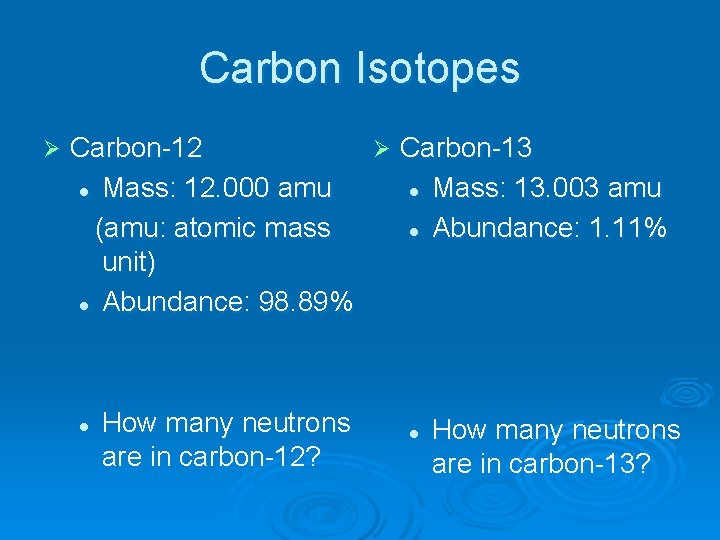Carbon Isotopes Ø Carbon-12 Ø Carbon-13 l Mass: 12. 000 amu l Mass: 13. 003 amu (amu: atomic mass l Abundance: 1. 11% unit) l Abundance: 98. 89% l How many neutrons are in carbon-12? l How many neutrons are in carbon-13?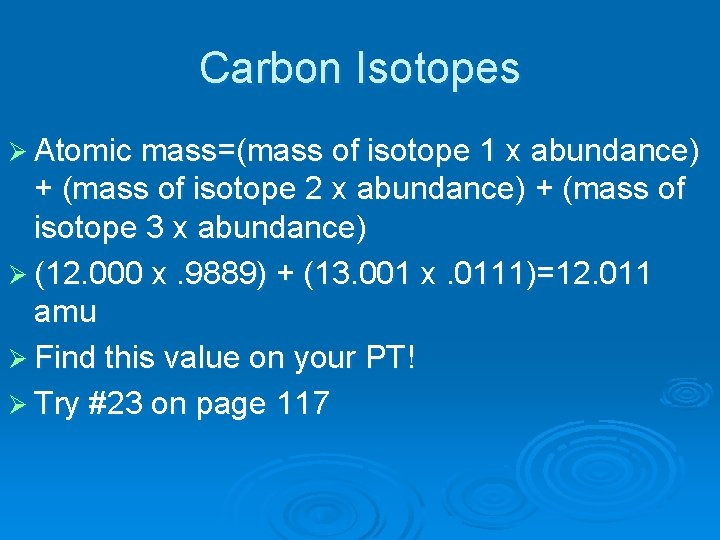Carbon Isotopes Ø Atomic mass=(mass of isotope 1 x abundance) + (mass of isotope 2 x abundance) + (mass of isotope 3 x abundance) Ø (12. 000 x. 9889) + (13. 001 x. 0111)=12. 011 amu Ø Find this value on your PT! Ø Try #23 on page 117Hydrogen Isotopes Hydrogen-1 Ø Name: protium Ø 1 e 1 p 0 n Hydrogen-2 Ø Name: deuterium Ø 1 e 1 p 1 n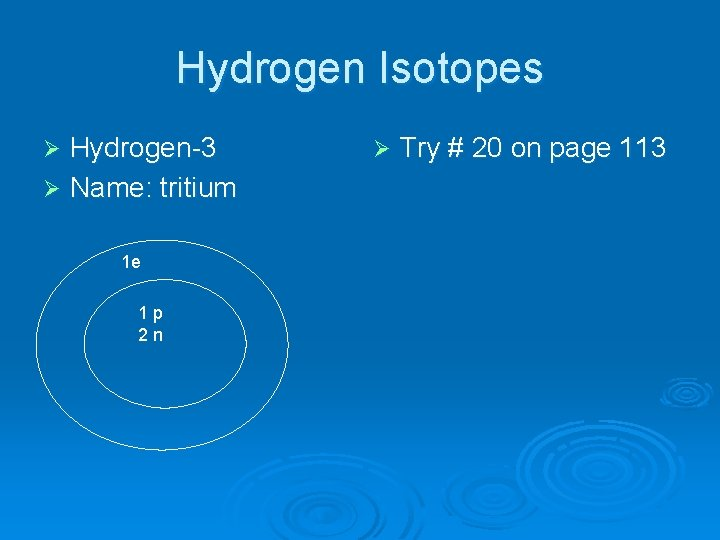Hydrogen Isotopes Hydrogen-3 Ø Name: tritium Ø 1 e 1 p 2 n Ø Try # 20 on page 113